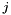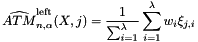#Boost C++ Libraries

...one of the most highly regarded and expertly designed C++ library projects in the world.

This is the documentation for an old version of boost. Click here for the latest Boost documentation.

## Struct template weighted_tail_variate_means_impl

boost::accumulators::impl::weighted_tail_variate_means_impl — Estimation of the absolute and relative weighted tail variate means (for both left and right tails)

## Synopsis

```// In header: <boost/accumulators/statistics/weighted_tail_variate_means.hpp>

template<typename Sample, typename Weight, typename Impl, typename LeftRight,
typename VariateType>
struct weighted_tail_variate_means_impl {
// types
typedef numeric::functional::average< Weight, Weight >::result_type                                                                       float_type;
typedef numeric::functional::average< typename numeric::functional::multiplies< VariateType, Weight >::result_type, Weight >::result_type array_type;
typedef iterator_range< typename array_type::iterator >                                                                                   result_type;

// construct/copy/destruct
weighted_tail_variate_means_impl(dont_care);

// public member functions
template<typename Args> result_type result(Args const &) const;
};```

## Description

For all-th variates associated to the

Equation 1.36.smallest samples (left tail) or the weighted mean of the

Equation 1.37.largest samples (right tail), the absolute weighted tail meansare computed and returned as an iterator range. Alternatively, the relative weighted tail meansare returned, which are the absolute weighted tail means normalized with the weighted (non-coherent) sample tail mean.

Equation 1.38.Equation 1.39.Equation 1.40.Equation 1.41.### `weighted_tail_variate_means_impl` public construct/copy/destruct

1. `weighted_tail_variate_means_impl(dont_care);`

### `weighted_tail_variate_means_impl` public member functions

1. `template<typename Args> result_type result(Args const & args) const;`
 Copyright © 2005, 2006 Eric Niebler Distributed under the Boost Software License, Version 1.0. (See accompanying file LICENSE_1_0.txt or copy at http://www.boost.org/LICENSE_1_0.txt)